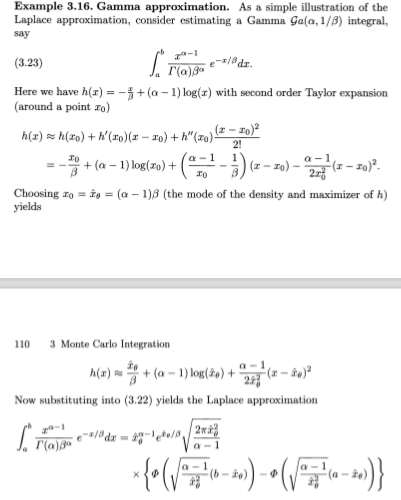Want to share your content on R-bloggers? click here if you have a blog, or here if you don't.I came across this question on X validated that wondered about one of our examples in Monte Carlo Statistical Methods. We have included a section on Laplace approximations in the Monte Carlo integration chapter, with a bit of reluctance on my side as this type of integral approximation does not directly connect to Monte Carlo methods. Even less in the case of the example as we aimed at replacing a coverage probability for a Gamma distribution with a formal Laplace approximation. Formal due to the lack of asymptotics, besides the length of the interval (a,b) which probability is approximated. Hence, on top of the typos, the point of the example is not crystal clear, in that it does not show much more than the step-function approximation to the function converges as the interval length gets to zero. For instance, using instead a flat approximation produces an almost as good approximation:

```>  xact(5,2,7,9)
 0.1933414
> laplace(5,2,7,9)
 0.1933507
> flat(5,2,7,9)
 0.1953668
```

What may be more surprising is the resilience of the approximation as the width of the interval increases:

```> xact(5,2,5,11)
 0.53366
> lapl(5,2,5,11)
 0.5354954
> plain(5,2,5,11)
 0.5861004